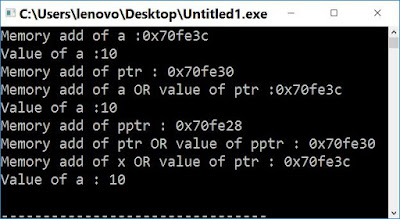## What is Pointer to pointer (Double Pointer)?

Till now, we were doing a single dereference. It means that there is a pointer that contains the memory address of a variable.

Pointer to pointer is a new concept in which we will do double dereferencing. It means that one pointer contains the memory address of another pointer or array of pointers in c. Pointer to pointer in c is declared as

int **pptr;

## How to initialize a pointer to pointer c++

Pointer to pointer always store the memory address of another pointer (Not Variable) as the below code will generate an error.

int x = 10
int **pptr=&x; //Error

We can use pointer to pointer as

int x = 10;
int *ptr = &x;
int **pptr = &ptr;

In the above code, using reference operator (&) memory address of variable x is assigned to a pointer ptr. It is important to understand that like a variable, when we declare a pointer, it also reserves memory equal to the size of 4 bytes regardless of its data type. In the last line, we are assigning the memory address of ptr to pptr. Using dereferencing, we can get the value and memory address of x, ptr, and pptr in the following ways:-

First way

cout<<"Mem add of x:";
cout<<&x;
cout<<"Value of x:"
cout<<x;

First line: Using reference operator memory address of variable x is obtained
Second line: Value of x is obtained by its name

Second way

cout<<"Mem add of ptr :";
cout<<&ptr;
cout<<"Mem add of x:";
cout<<ptr;
cout<<"Value of x :";
cout<<*ptr;

First line: Address of memory spaces reserved by ptr is obtained using reference operator
Second line: Address, where ptr points to, is obtained OR value of ptr by its name
Third line: Value of x is obtained using a single dereference operator

Third Way

cout<<"Mem add of pptr:";
cout<<&pptr;
cout<<"Mem add of ptr:";
cout<<pptr;
cout<<"Mem add of x:";
cout<<*pptr;
cout<<"Value of x :";
cout<<**pptr;

First line: Address of memory spaces reserved by pptr is obtained using & operator
Second line: Memory address of ptr where pptr points to is obtained OR value of pptr by its name
Third line: Value of pptr (which is the memory address of ptr) is obtained using single deference operator
Forth line: Value of x is obtained using double deference operatorPointer to pointer C++
OutputPointer to pointer c++ output

### Post a Comment

Previous Post Next Post